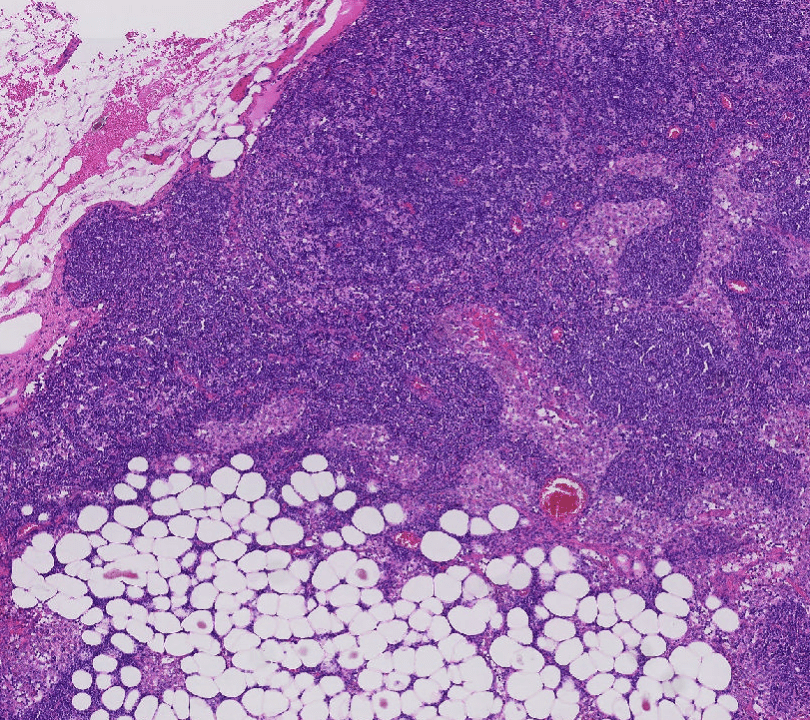# Process Big Images Efficiently Using Partial Images or Lower Resolutions

This example shows how to process a big image quickly using two strategies that enable computations on smaller representative samples of the high-resolution image.

Processing big images can be time consuming. This makes iterative development of algorithms prohibitively expensive. There are two common ways to shorten the feedback cycle: iterate on a lower resolution image or iterate on a partial region of the big image. This example demonstrates both of these approaches for creating a segmentation mask for a big image.

If you have Parallel Computing Toolbox™ installed, then you can further accelerate the processing by using multiple workers.

Create a `bigimage` using a modified version of image "tumor_091.tif" from the CAMELYON16 data set. The original image is a training image of a lymph node containing tumor tissue. The original image has eight resolution levels, and the finest level has resolution 53760-by-61440. The modified image has only three coarse resolution levels. The spatial referencing of the modified image has been adjusted to enforce a consistent aspect ratio and to register features at each level.

```bim = bigimage('tumor_091R.tif'); ```

Display the big image by using the `bigimageshow` function.

```bigimageshow(bim); ```### Accelerate Processing Using Lower Resolution Image

Many big images contain multiple resolution levels, including coarse lower resolution versions of the finest high-resolution image. In general, the distribution of individual pixel values should be roughly equal across all the levels. Leveraging this assumption, you can compute global statistics at a coarse level and then use the statistics to process the finer levels.

Extract the image at the coarsest level, then convert the image to grayscale.

```imLowRes = getFullLevel(bim,bim.CoarsestResolutionLevel); imLowResGray = rgb2gray(imLowRes); ```

Threshold the image into two classes and display the result.

```thresh = graythresh(imLowResGray); imLowResQuant = imbinarize(imLowResGray,thresh); imshow(imLowResQuant) ```Validate on the largest image. Negate the result to obtain a mask for the stained region.

```bq = apply(bim,bim.FinestResolutionLevel, ... @(im)~imbinarize(rgb2gray(im),thresh)); ```

Visualize the result at the finest level.

```bigimageshow(bq,'CDataMapping','scaled'); ```### Accelerate Processing Using Using Partial Regions of Big Image

Another approach while working with large images is to extract a smaller region with features of interest. You can compute statistics from the ROI and then use the statistics to process the entire high-resolution image.

```% Zoom in on a region of interest. bigimageshow(bim); xlim([2400,3300]) ylim([900 1700]) ```Extract the region being shown from the finest level.

```xrange = xlim; yrange = ylim; imRegion = bim.getRegion(1,[xrange(1),yrange(1)],[xrange(2),yrange(2)]); imshow(imRegion); ```Prototype with this region then display the results.

```imRegionGray = rgb2gray(imRegion); thresh = graythresh(imRegionGray); imLowResQuant = ~imbinarize(imRegionGray,thresh); imshow(imLowResQuant) ```Validate on the full big image and display the results.

```bq = apply(bim, bim.FinestResolutionLevel,... @(im)~imbinarize(rgb2gray(im), thresh)); bigimageshow(bq,'CDataMapping','scaled'); ```### Accelerate Processing Using Parallel Computing Toolbox

If you have the Parallel Computing Toolbox™ installed, then you can distribute the processing across multiple workers to accelerate the processing. To try processing the image in parallel, set the `runInParallel` variable to `true`.

```runInParallel = false; if runInParallel % Open a pool p = gcp; % Ensure workers are on the same folder as the file to be able to % access it using just the relative path sourceDir = fileparts(which('tumor_091R.tif')); spmd cd(sourceDir) end % Run in parallel bq = apply(bim,bim.FinestResolutionLevel, ... @(im)~imbinarize(rgb2gray(im),thresh),'UseParallel',true); end ```